# Excel : Goal Seek

This tutorial explains how to use Goal Seek in Excel. It includes explanation of this feature with several practical examples.

What is Goal Seek?

Goal Seek is an inbuilt excel utility that solves an equation and helps you to answer this question "What would be the input value to get the desired result".

It asks us to input three parameters :
1. Formula in a cell
2. Target / Desired Value
3. Which cell to change
Let's explain it with case studies :

Case Study I

Suppose you have a data set in which there are some missing values. You need to calculate the number of non-missing values required if you need to maintain 70% of non-missing values in your data. See the snapshot of data shown in the image below-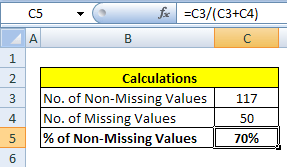Excel : Goal Seek

Data Preparation for Goal Seek

The following are the steps to prepare data before running Goal Seek.
1. Type the text 'No. of Non-Missing Values' in cell B3 and enter any dummy value (let's say 117) corresponding to it in cell C3. We'll calculate the exact value in the following steps.
2. Enter the text 'No. of Missing Values' in cell B4 and the actual figure (let's say 50) in cell C4.
3. Type '% of Non-Missing Values' in cell B5 and enter the following formula in cell C5.
4. C3/(C3+C4)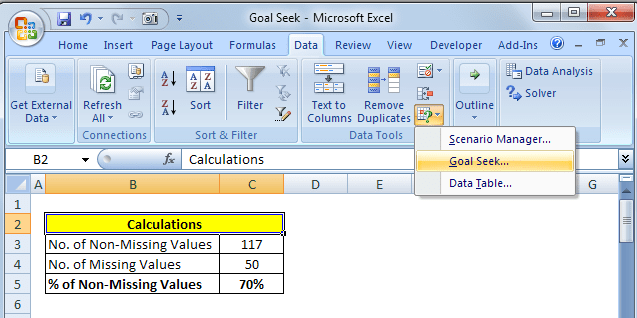Goal Seek Menu
5. Go to the 'Data' tab and click on 'What-If Analysis' button and select Goal Seek under it. See and follow the instructions shown in the image above.
6. When you click on Goal Seek button, it will open a box in which you need to provide cell reference and target value. The explanation is provided below -

Q. What would be the number of non-missing values if we want to get 60% of non-missing values?Goal Seek Parameters
Set cell :  Cell reference wherein formula is entered. In this case, give cell reference of C5.

To value : Target/ Desired value. In this case, it is 60%. Make sure you enter % after 60. You can also type 0.6 instead of 60%

By changing cell : reference to the cell that has the value you want to change. In this example, it is reference of cell C3.

Once you have filled the above 3 inputs, click on 'Ok' button and then Excel would find solution for you and show you the result in cell C3Goal Seek Solution
Solution : We require total 75 non-missing values to have 60% non-missing percentage.

How can we do it without Goal Seek?

We can calculate it through building mathematical equations -

Step I : C3 / (C3+C4) = 0.6

Step II : (C3 * 0.6) + ( C4 * 0.6) = C3

Step III : (1 - 0.6) * C3 = C4*0.6

Step IV :  C3 = (C4*0.6) / (1 - 0.6)

If you enter equation of step IV in the formula =(C4*0.6)/(1-0.6), it would return a desired value.

Is the above solution correct?

Answer is NO. It is because the number of missing values are dependent on non-missing values. Both makes a complete dataset. For example, you have a data set which comprises of total 150 records. If you fill 15 missing records to some value, it would increase the number of non-missing records by 15 and the number of missing records would accordingly fall by 15 records. To workaround this issue, we need to include total number of records as a constraint. See the solution below.

Add a Constraint in Goal Seek

Since we have assumed 150 total number of rows/records in the dataset, we can set this constraint in the formula.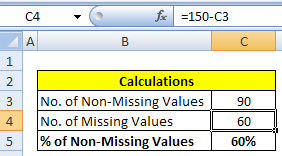Goal Seek Constraint
1. Enter a formula =150-C3 in cell C4 (As shown in the image above)
2. Run Goal Seek Analysis again (Follow the instructions below)Goal Seek : Instructions
In this case, it returns a solution which also considers the number of cases in data and make sure it's always equal to 150.

Important Points
1. The cell reference in Set cell: box must contain a formula. Otherwise, it would return an error.
2. You cannot give cell reference in 'To value:' box. You need to enter a value.
3. The cell reference in 'By changing cell:' cannot contain a formula. It must be a typed value.

Case Study II

Suppose you want to buy a luxury car which costs \$150,000. To finance it, you take out a car loan from a bank for \$150,00 at 14% interest rate. You can pay maximum \$4,000 per month in instalment. You need to figure out how many periods you need to pay monthly payment?
You can use PMT() function to calculate the payment for a loan.
Loan Instalment = PMT(Interest Rate, No. of Payments, Loan Amount)

In this case, we need to calculate 'No. of Payments' which is a parameter in the function itself.

Data Preparation

1. Enter figures of Loan Amount, No. of Payments (Monthly) and Annual Interest Rate from cell C3 through C5. Put any dummy value against No. of Payments. We'll calculate the correct value later.
2.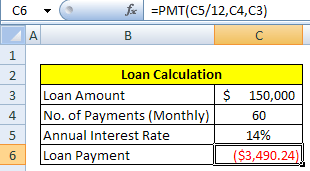Goal Seek - Loan Payment
3. Enter formula =PMT(C5/12,C4,C3) in cell C6 (Refer the image above). Since C5 is annual interest rate, we need to divide it by 12
4. Go to Data tab and then click on What-If Analysis and select Goal Seek
5. In Set cell:, enter reference of cell C6.
6. In To value:, enter -4000. Make sure it is negative since it is a payment.
7. In By changing cell:, give cell reference of C4 ( Refer figure 2)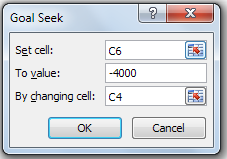Figure 2

Solution : Goal Seek found the solution and it is required to pay 50 monthly payments when \$4000 is paid monthly in installment.

Endnotes

Goal Seek is very helpful for quickly solving any financial and statistical formula for a single unknown value. It saves a lot of time when it is difficult to derive a formula to calculate unknown value. As shown in the second case study, it takes time to transform equation of loan payment and calculate the terms without using Goal Seek. If you have a complex problem which contains multiple equations and multiple constraints and you are asked to find the optimal solution, you should look for Excel's Solver Add-In instead of Goal Seek. Excel Solver is a powerful method to solve optimization problem.

Related Posts1.